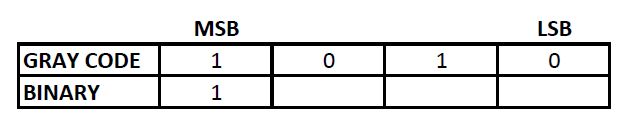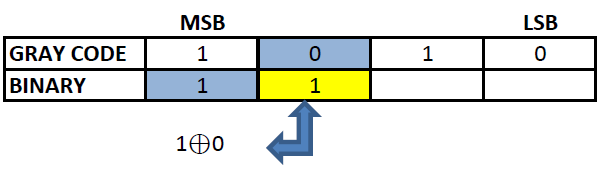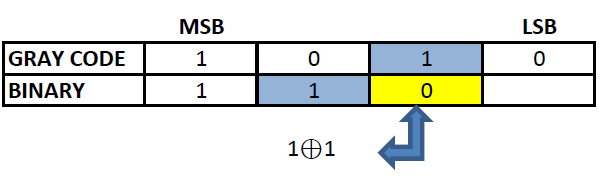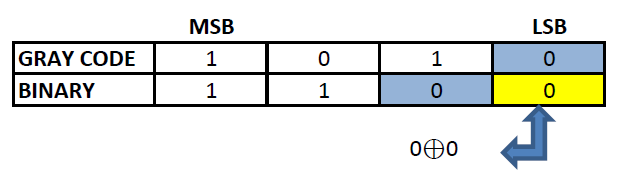# How to convert gray code to hexadecimal

## Gray code

Gray code, also known as reflected binary code, is a code having digits 0 and 1. Gray code do not have place value for its digits. Any successive codes in Gray code system have only one bit changes.

Hexadecimal number system uses 16 different symbols to represent a numeric value. It uses numbers 0 to 9 and alphabets A to F for representation. . The place value of each digits of an hexadecimal number varies as the whole number powers of 16 starting from the right (Least Significant Digit). The first single digit number in hexadecimal system is 0 and the last is F. Similarly, the first two digit hexadecimal number is 10 and the last is FF and so on. It is used as an alternative for binary numbers by developers and programmers.

## Binary number

Binary numbering system uses only two symbols 0 and 1. Each digits of a binary number is referred to as bits. Binary system is also known as base -2 system. Each digit is represented by the increasing power of 2 from the LSB (Least Significant Bit). Binary system is the heart of digital electronics and is used for information flow. In digital electronics, 0 and 1 are used to denote logic states, high and low. Arithmetic operations are also possible in binary system.

## Gray code to hexadecimal conversion

Gray code cannot be directly converted to hexadecimal. For converting gray code to hexadecimal, we have to convert the gray code to binary first and then convert the binary code to hexadecimal.

Gray to hexadecimal conversion can be well understood with an example.

### Let us convert 1010 from gray code to hexadecimal value:

To convert 1010 to hexadecimal, we have to convert 1010 to binary first.
Step 1: The MSB (Most Significant Bit) of a binary and gray code will be the same.Step 2: The next digit of binary will be the EXOR of the MSB of binary and the second MSB of the gray code.Step 3: Similarly EXOR the digit obtained in the previous step and the third MSB of gray code to obtain the third MSB of binary.Step 4: Repeat the previous step till the LSB of gray code is found.### The Binary corresponding to gray code 1010 is 1100. Now this binary code can be converted to hexadecimal.

11002 = C16
Therefore, Gray code 1010 = C16